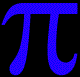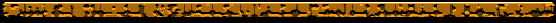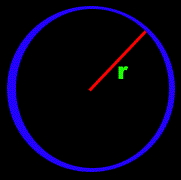The Validity of PiPurpose: Since it's discovery by the father of Geometry, Euclid, Pi has been the cornerstone of  classical physics and math. Derived from circles, Scientific AmeriKen will repeat the processes used to find this figure, and hopefully prove the validity of Pi.Hypothesis: Since the current value of Pi seems to work for everything, Scientific AmeriKen will hope that the results of the experiment will yield Pi having a value of 22/7 or roughly 3.14Equipment: Needed for this experiment is one circle, one cylindrical object capable of having it's volume measured, a measuring tape, pen, paper, and a calculator is optional.Procedure:  This first step is to measure the circumfrance and the radius of the circle. Circumfrance is the distance around the outside of the circle, (blue line) the radiusis the section going from the center to the outside, labeled with a red line. The formula given for the circumfrance of a circle is given by C = 2(pi)r, By dividing the measured circumfrance by twice the value of the radius, the value of Pi should be determined. The next step is to repeat this experiment with the cylinder. Measurements for this require measuring the radius, and the height of the cylinder, as well as finding out the total volume to object holds. The formula then becomes, V=(pi)r^2h, therefore, dividing the volume by the square of the radius times the height will give the value of pi.Results:

Circumferance Experiments

 Object Circumfrance Diameter Experimental Value of Pi Roll of Tape 34.95 cm 10.95 cm 3.19 Record 94.49 cm 32.66 cm 2.89

 Experimental  Ave. Value of Pi True value of Pi Percent Error 3.04 3.142857143 3.27%
Volume Experiments

 Object Given Volume Measured Height Measured Radius Exp. Value of Pi Soda Can 354.72 Cubic cm 11.76 cm 3.19 cm 2.97 Bottle of Whiteout 17.74 Cubic cm 4.45 cm 1.15 cm 3.01

 Experimental Ave. value of Pi True Value of Pi Percent Error 2.99 3.14 4.78%Conclusion: Based on the percent error of roughly 3-4% it is safe to say that Pi is an adequate approximation in dealing with circles, and furthermore, it would be tough to argue against all of science that the value of Pi is wrong based on it being only 3% off. Therefore the hypothesis is proven true, however, Scientific AmeriKen would like to state that just because the constant of Pi has been scientifically proven does not mean that other constants such as c (speed of light) e (the value of the natural log) and many others are not exempt from the scrutiny of Scientific AmeriKen!

 Scientific AmeriKen would wish to make a dedication to several  contributers, Brian Kobashikawa, for  assistance of musical selections, as well as some pieces of his own, Brad Hutcheson, and "Dr. John" for additional reasearch they have done on their own.  Thanks!
Stratus by Brian Kobashikawa
Back to Scientific AmeriKen!